# Problems on H.C.F and L.C.M - Quantitative Aptitude (MCQ) questions

Dear Readers, Welcome to Quantitative Aptitude Problems on H.C.F and L.C.M questions and answers with explanation. These Problems on H.C.F and L.C.M solved examples with shortcuts and tricks will help you learn and practice for your Placement Test and competitive exams like Bank PO, IBPS PO, SBI PO, RRB PO, RBI Assistant, LIC,SSC, MBA - MAT, XAT, CAT, NMAT, UPSC, NET etc.

After practicing these tricky Problems on H.C.F and L.C.M multiple choice questions, you will be exam ready to deal with any objective type questions.

1)   Greatest Common Divisor of two numbers is 8 while their Least Common Multiple is 144. Find the other number if one number is 16.
- Published on 03 May 17

a. 108
b. 96
c. 72
d. 36
 Answer  Explanation ANSWER: 72 Explanation: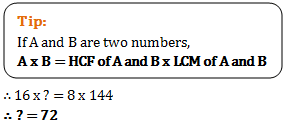2)   LCM of two numbers is 138. But their GCD is 23. The numbers are in a ratio 1:6. Which is the largest number amongst the two?
- Published on 03 May 17

a. 46
b. 138
c. 69
d. 23
 Answer  Explanation ANSWER: 138 Explanation: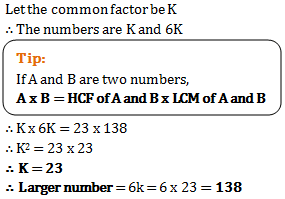3)   The least common multiple of two numbers is 168 and highest common factor of them is 12. If the difference between the numbers is 60, what is the sum of the numbers?
- Published on 12 Apr 17

a. 108
b. 96
c. 122
d. 144
 Answer  Explanation ANSWER: 108 Explanation: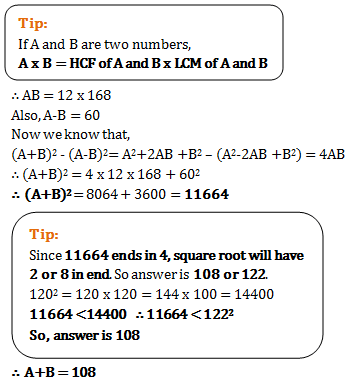4)   If least common multiple of two numbers is 225 and the highest common factor is 5 then find the numbers when one of the numbers is 25?
- Published on 11 Apr 17

a. 75
b. 65
c. 15
d. 45
 Answer  Explanation ANSWER: 45 Explanation: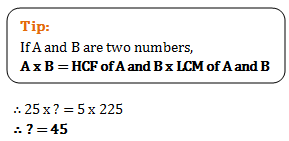5)   The greatest number of four digits which is divisible by 15, 25, 40, 75 is
- Published on 07 Jul 17

a. 600
b. 9000
c. 9600
d. 9400
 Answer  Explanation ANSWER: 9600 Explanation: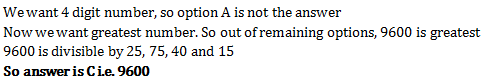6)   What is the least number which when divided by the numbers 3, 5, 6, 8, 10 and 12 leaves in each case a remainder 2 but which when divided by 13 leaves no remainder?
- Published on 07 Jul 17

a. 312
b. 962
c. 1586
d. 1562
 Answer  Explanation ANSWER: 1586 Explanation: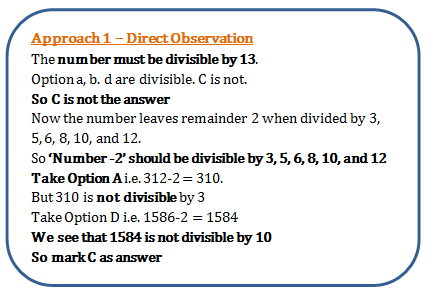7)   When a number is divided by 893 the remainder is 193. What will be the remainder when it is divided by 47?
- Published on 07 Jul 17

a. 19
b. 5
c. 33
d. 23
 Answer  Explanation ANSWER: 5 Explanation: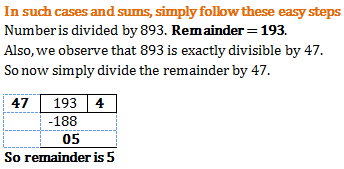8)   The sum of two numbers is 156 and their HCF is 13. The numbers of such number pairs is
- Published on 07 Jul 17

a. 2
b. 5
c. 4
d. 3
 Answer  Explanation ANSWER: 2 Explanation: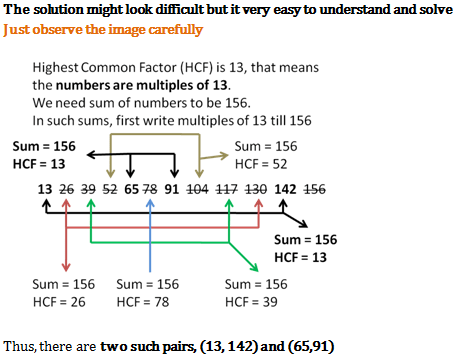9)   The L.C.M. of two numbers is 14560 and their H.C.F. is 13. If one of them is 416, the other is
- Published on 07 Jul 17

a. 460
b. 455
c. 450
d. 445
 Answer  Explanation ANSWER: 455 Explanation: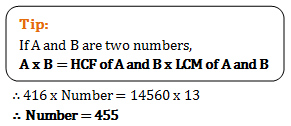10)   The greatest length of the scale that can measure exactly 30 cm, 90 cm, 1 m 20 cm and 1 m 35 cm lengths is
- Published on 05 Apr 17

a. 5 cm
b. 10 cm
c. 15cm
d. 30 cm
 Answer  Explanation ANSWER: 15cm Explanation: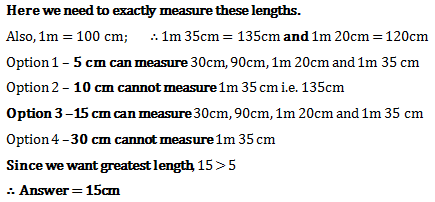1 2 3 4